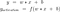# Activation Functions (Part 1)

An activation is a function applied to the output of a neuron that allows it to learn more complex functions as we go deeper in a neural network. They can also be thought of as mapping to modify the range of a function. For example we could use an activation function to ensure that all our outputs lie between 0 and 1, or between -1 and 1.Figure 1: Expression for the output of a neuron, followed by applying an activation function
• The first equation in Figure 1 is the formula used to calculate the output of a neuron. The equation takes the form of a straight line y = mx + c

# Basics of Vectors and Coordinate Space

Vectors and Coordinate spaces are topics often overlooked as they seem very self explanatory, and easy to understand, from a point of view of mathematical formulas. However, unless we understand intuitively the impact of various vector operations on coordinate spaces, it becomes a lot more difficult to grasp concepts like Linear Transformations or Jacobians. In this post, I hope to lay the ground with the basics of vectors, coordinate spaces and projections, and build on these concepts in future posts.

The figure above is the basic 2 Dimensional coordinate system we are used to seeing. The X axis is horizontal…

# Creating a custom Dataset and Dataloader in Pytorch

Training a deep learning model requires us to convert the data into the format that can be processed by the model. For example the model might require images with a width of 512, a height of 512, but the data we collected contains images with a width of 1280, and a height of 720. We therefore need some way to be able to convert the available data we have, into the exact format required by the model.

A dataloader in simple terms is a function that iterates through all our available data and returns it in the form of batches…

# Non Max Suppression (NMS)

What is Non Max Suppression, and why is it used?

Non max suppression is a technique used mainly in object detection that aims at selecting the best bounding box out of a set of overlapping boxes. In the following image, the aim of non max suppression would be to remove the yellow, and blue boxes, so that we are left with only the green box.

Procedure for calculating NMS:

To get an overview of what a bounding box is, and what IOU means, I have made two posts on the same.(Bounding Box, and IOU). The terms and parameters described in…

# IOU (Intersection over Union)

What is IOU and where is it used?

• IOU(Intersection over Union) is a term used to describe the extent of overlap of two boxes. The greater the region of overlap, the greater the IOU.
• IOU is mainly used in applications related to object detection, where we train a model to output a box that fits perfectly around an object. For example in the image below, we have a green box, and a blue box. The green box represents the correct box, and the blue box represents the prediction from our model. The aim of this model would be to keep…

# Basics of Bounding Boxes

What is a bounding box?

A bounding box in essence, is a rectangle that surrounds an object, that specifies its position, class(eg: car, person) and confidence(how likely it is to be at that location). Bounding boxes are mainly used in the task of object detection, where the aim is identifying the position and type of multiple objects in the image. For example, if you look at the image below, the green rectangle is a bounding box that describes where in the image, the car lies.